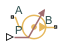# Pressure Source (TL)

Generate constant or time-varying pressure differential

•Libraries:
Simscape / Foundation Library / Thermal Liquid / Sources

## Description

The Pressure Source (TL) block represents an ideal mechanical energy source in a thermal liquid network. The source can maintain the specified pressure differential across its ports regardless of the flow rate through the source. There is no flow resistance and no heat exchange with the environment.

Ports A and B represent the source inlet and outlet. The input physical signal at port P specifies the pressure differential. Alternatively, you can specify a constant pressure differential as a block parameter. A positive pressure differential causes the pressure at port B to be greater than the pressure at port A.

The energy balance at the source is a function of the energy flow rates through ports A and B and the work done on the fluid:

`${\varphi }_{A}+{\varphi }_{B}+{\varphi }_{work}=0,$`

where:

• ϕA is the energy flow rate into the source through port A.

• ϕB is the energy flow rate into the source through port B.

• ϕwork is the isentropic work done on the fluid.

The isentropic work term is

`${\varphi }_{work}=\frac{\stackrel{˙}{m}\left({p}_{B}-{p}_{A}\right)}{{\rho }_{avg}},$`

where:

• ϕwork is the isentropic work done on the thermal liquid.

• pA is the pressure at port A.

• pB is the pressure at port B.

• ρavg is the average liquid density,

`${\rho }_{avg}=\frac{{\rho }_{A}+{\rho }_{B}}{2}\text{.}$`

### Assumptions and Limitations

• There are no irreversible losses.

• There is no heat exchange with the environment.

## Ports

### Input

expand all

Input physical signal that specifies the pressure differential across the source.

#### Dependencies

To enable this port, set the Source type parameter to `Controlled`.

### Conserving

expand all

Thermal liquid conserving port. A positive pressure differential causes the pressure at port B to be greater than the pressure at port A.

Thermal liquid conserving port. A positive pressure differential causes the pressure at port B to be greater than the pressure at port A.

## Parameters

expand all

Select whether the pressure differential generated by the source can change during simulation:

• `Controlled` — The pressure differential is variable, controlled by an input physical signal. Selecting this option exposes the input port P.

• `Constant` — The pressure differential is constant during simulation, specified by a block parameter. Selecting this option enables the Pressure differential parameter.

Desired pressure differential across the ports of the source.

#### Dependencies

To enable this parameter, set Source type to `Constant`.

Select whether the source performs work on the fluid flow:

• `Isentropic` — The source performs isentropic work on the fluid to maintain the specified pressure differential. Use this option to represent an idealized pump or compressor and properly account for the energy input and output, especially in closed-loop systems.

• `None` — The source performs no work on the flow, neither adding nor removing power, regardless of the pressure differential produced by the source. Use this option to set up the desired flow condition upstream of the system, without affecting the temperature of the flow.

Area normal to the direction of flow at the source inlet and outlet. The two cross-sectional areas are assumed identical.

## Version History

Introduced in R2013b

expand all